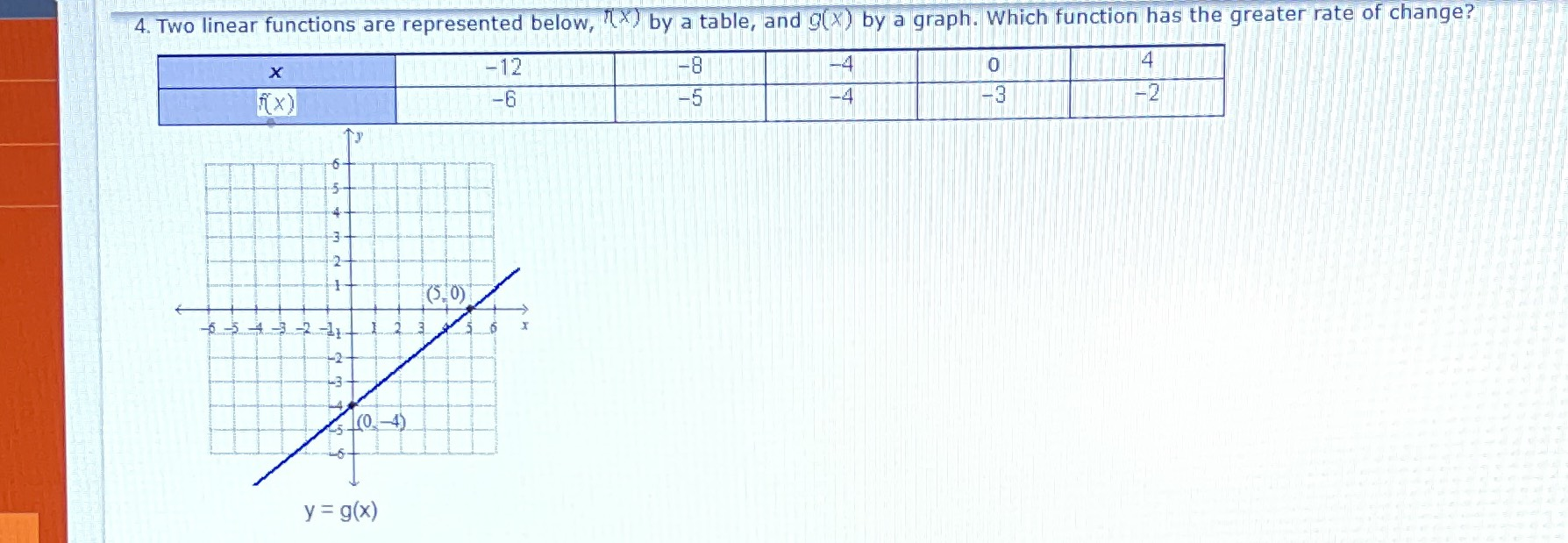### ¿Todavía tienes preguntas de matemáticas?

Pregunte a nuestros tutores expertos
Algebra
Pregunta4. Two linear functions are represented below, $$f ( x )$$ by a table, and $$g ( x )$$ by a graph. Which function has the greater rate of change?

F(X)=$$\frac{1}{4}x- 3$$
g(x)=$$\frac{4}{5}x- 4$$